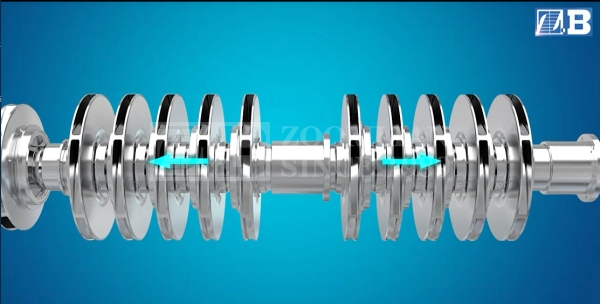Focus on centrifugal pump manufacturing since 1989
24小时服务热线：sales@zoomlian.com

## Axial thrust_Changsha Zoomlian Pump Co.,Ltd

Axial thrust
The axial thrust is the resultant force of all the axial forces (F) acting on the pump rotor.

Axial thrust: the axial force in a single-stage centrifugal pumpThe axial force acting on the rotor in a single-stage centrifugal pump

The impeller axial force (F1) is the difference between the axial force on the impeller cover on the discharge side (Fd) and the suction side (Fs)
F1 = Fd – Fs

Momentum (FJ) is a force that constantly acts on a fluid in a defined space (see Principles of Conservation of Momentum, Fluid Mechanics). Calculated as follows:
FJ =ρ·Q·ΔVax

Q flow
ρ Density of processing fluid
ΔVax The difference between the axial component of the absolute velocity at the inlet and outlet of the impeller
On the relevant shaft cross section, the resultant pressure Ass produced by the static pressure (ss) upstream and downstream of the shaft seal: FWd = AWd·ΔpWd
Special axial forces, such as when the eddy current conditions in the gap between the impeller and the casing (side gap) change during start-up (see Disc Friction)
Other axial forces, such as the force of the rotor counterweight (FW) on non-horizontal centrifugal pumps or the magnetic attraction in the electric motor (Fmech), such as in closed pumps
The axial thrust component (F1 + FJ) of a hydraulically unbalanced closed impeller (that is, with a suction side shield) is:

The axial thrust coefficient basically depends on the specific speed (ns). For radial flow and mixed flow impellers, the following equation applies to the range of 6 <ns <130 rpm:

α= 0,5·(Dsp / D2m) 3 + 0,09≈0,1-0,3

The diameter of the controlled gap seal on the suction side impeller cover

Axial thrust: unbalanced impeller design with tapered impeller outlet area

This formula applies to flow rates (Q) from 0.8 (Qopt) to 1.0 (Qopt) and gap width s = 0.1 mm. If the gap width is doubled, α increases by 8%.

For multi-stage pumps with diffusers (such as boiler feed pumps), the axial impeller force (F1) depends largely on the axial position of the impeller relative to the diffuser. For an open radial impeller without a shroud on the suction side, the axial force (Fs) is much lower than the closed impeller, which means that the axial impeller force (F1) is higher.

An open impeller with cutouts in the impeller shroud between adjacent impeller blades produces less pressure (Fd), so the axial force (F1) is lower than that of an impeller with a complete exhaust side cover.

For an axial propeller, the axial thrust coefficient (α) is almost equal to the degree of reaction (rth). Then you can use the outer diameter (OD) of the propeller to roughly calculate the axial thrust:

For geometrically similar pumps, the following ratio applies to the F1 component of the axial thrust (see Figure 1 Axial thrust) at the defined speed (n) and maximum impeller diameter (D2):

The rotation of the fluid processed in the gap between the impeller and the casing in the discharge side and the suction side has a great influence on the axial pressure (Fd) and (Fs). The average angular velocity (see Rotation Speed) of the processed rotating fluid reaches approx. Half the speed of the impeller.

In addition, due to the Coriolis acceleration, the inwardly directed gap flow (side gap) in the suction side (ie, outer) gap between the impeller and the casing further increases the side gap turbulence. In the discharge side (ie, internal) gap of a multistage pump where the impeller is not hydraulically balanced, the process is reversed because the gap gap faces outward. The scroll movement decelerates, causing the axial force Fd to increase, which leads to an increase in F1.

During the start-up process, the axial impeller force is higher than in the steady state operation, because during the start-up process, due to the disc friction caused by the action of the impeller guard or the braking action of the fixing device, the processed fluid starts to be slow Rotate the shell surface.

◎欢迎参与讨论，请在这里发表您的看法、交流您的观点。

Need to assist with pumps?